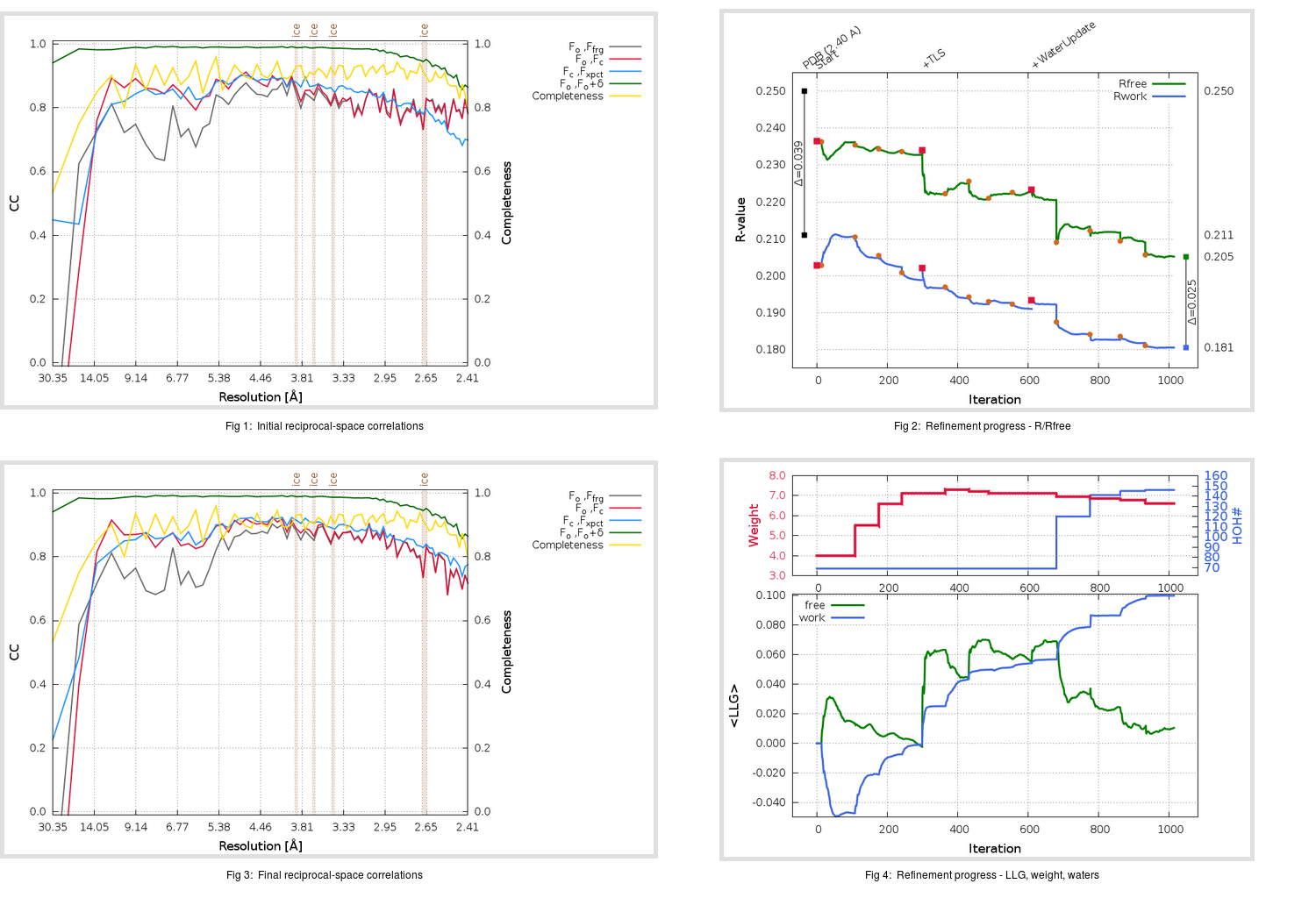Content:

## Deposited

` `
 Date deposited Date data collection Resolution R, Rfree 20110629 20090927 2.40 0.2090 0.2500

Molprobity (CCP4 7.0 version) summary:

```Ramachandran outliers =   0.65 %
favored =  95.10 %
Rotamer outliers      =   6.30 %
C-beta deviations     =     1
Clashscore            =   7.48
RMS(bonds)            =   0.0131
RMS(angles)           =   1.41
MolProbity score      =   2.36
Resolution            =   2.40
R-work                =   0.2090
R-free                =   0.2500
```

```Number of waters      =    69

<B> (all atoms) =   38.22 ( sd =   11.90 ) for       2504 non-hydrogen atoms
<B>   (protein) =   38.03 ( sd =   11.44 ) for       2423 non-hydrogen atoms
<B>     (water) =   36.95 ( sd =    8.59 ) for         69 non-hydrogen atoms
<B>    (others) =   96.59 ( sd =    5.17 ) for         12 non-hydrogen atoms

B min/max       (all non-hydrogen atoms) =   15.15 /  117.26
B min/max   (protein non-hydrogen atoms) =   15.69 /  117.26
B min/max     (water non-hydrogen atoms) =   15.15 /   60.65
B min/max     (other non-hydrogen atoms) =   33.84 /  106.08
```

## BUSTER (re-)refinement

` `

Molprobity (CCP4 7.0 version) summary:

```Ramachandran outliers =   0.00 %
favored =  96.41 %
Rotamer outliers      =   6.30 %
C-beta deviations     =     1
Clashscore            =   5.40
RMS(bonds)            =   0.0117
RMS(angles)           =   1.61
MolProbity score      =   2.14
Resolution            =   2.40
R-work                =   0.1807
R-free                =   0.2053
```

```Number of waters      =   146

<B> (all atoms) =   46.40 ( sd =   11.73 ) for       2581 non-hydrogen atoms
<B>   (protein) =   46.02 ( sd =   11.59 ) for       2423 non-hydrogen atoms
<B>     (water) =   50.62 ( sd =   10.79 ) for        146 non-hydrogen atoms
<B>    (others) =   77.16 ( sd =    2.07 ) for         12 non-hydrogen atoms

B min/max       (all non-hydrogen atoms) =   23.34 /  100.01
B min/max   (protein non-hydrogen atoms) =   26.41 /  100.01
B min/max     (water non-hydrogen atoms) =   23.34 /   84.37
B min/max     (other non-hydrogen atoms) =   33.84 /   80.35
```

Refinement progression:Results:

` `
 File Remark 3SNB_aB_refine.01_03_refine.pdb.gz exact refinement commands are in header 3SNB_aB_refine.01_03_refine.mtz.gz including original deposited data and several re-refinement map coefficients 3SNB_aB_refine.01_03_BUSTER_model.cif.gz including any non-standard compound restraints 3SNB_aB_refine.01_03_BUSTER_refln.cif.gz